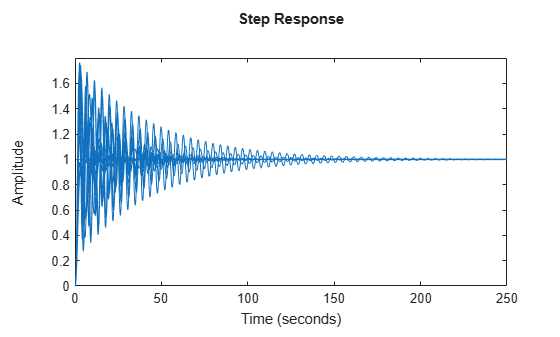# Model Gain and Phase Uncertainty in Feedback Loops

This example shows how to model gain and phase uncertainty in feedback loops using the `umargin` control design block. The example also shows how to check a feedback loop for robust stability against such uncertainty.

### Modeling Gain and Phase Uncertainty

Consider a SISO feedback loop with open-loop transfer function

$\mathit{L}=\frac{3.5}{{\mathit{s}}^{3}+{2\mathit{s}}^{2}+3\mathit{s}}$.

```L = tf(3.5,[1 2 3 0]); bode(L) grid on```Due to plant uncertainty and other sources of variability, the loop gain and phase are subject to fluctuations. In general, you can quantify the amount of uncertainty through experimenting on your system, or approximate it based on insight or experience. For this example, suppose that the open-loop gain can increase or decrease by 50%, and the phase by ±30°. You can use the `umargin` block to model such uncertainty. `umargin` represents the variation as an uncertain multiplicative factor `F` with nominal value 1. The set of values F can take captures the gain and phase uncertainty you specify.

To create the `umargin` block, use `getDGM` to compute the smallest uncertainty disk that captures the gain and phase variation you want to represent. Use the output of `getDGM` to create F.

```DGM = getDGM(1.5,30,'tight'); F = umargin('F',DGM)```
```Uncertain gain/phase "F" with relative gain change in [0.472,1.5] and phase change of ±30 degrees. ```

Visualize F to see the range of values taken by this factor (right) and the range of gain and phase variations it models by `F` (left).

`plot(F)`The plots show that the gain can vary between 47% and 150% of its nominal value (assuming no phase variation) and the phase can vary by ±30° (assuming no gain variation). When both gain and phase vary, their variation stays inside the shaded region in the left plot.

The uncertainty F multiplies the open-loop response, yielding a closed-loop system as in the following diagram.Incorporate this uncertainty into the closed-loop model.

`T = feedback(L*F,1)`
```Uncertain continuous-time state-space model with 1 outputs, 1 inputs, 3 states. The model uncertainty consists of the following blocks: F: Uncertain gain/phase, gain × [0.472,1.5], phase ± 30 deg, 1 occurrences Type "T.NominalValue" to see the nominal value and "T.Uncertainty" to interact with the uncertain elements. ```

The result is an uncertain state-space (`uss`) model of the closed-loop system containing the uncertain block F. In general the open-loop gain can contain other uncertain blocks too.

### Robustness Analysis

Sampling the uncertainty and plotting the closed-loop step response suggest poor robustness to such gain/phase variations.

```clf rng default step(T)```To quantify this poor robustness, use `robstab` to gauge the robust stability margin for the specified uncertainty.

`SM = robstab(T)`
```SM = struct with fields: LowerBound: 0.8303 UpperBound: 0.8319 CriticalFrequency: 1.4482 ```

The robust stability margin is only 0.83, meaning that the feedback loop can only withstand 83% of the specified uncertainty. The factor 0.83 is in normalized units. To translate this value into an actual safe range of gain and phase variations, use `uscale`. This command takes a modeled uncertainty disk and a scaling factor, and converts it into a new uncertainty disk.

`Fsafe = uscale(F,0.83)`
```Uncertain gain/phase "F" with relative gain change in [0.564,1.42] and phase change of ±24.8 degrees. ```

The display shows that 83% of the uncertainty specified in `F` (and therefore in `L`) amounts to gain variation between 56% and 142% of the nominal value, and phase variation of ±25°. Plot the disk `Fsafe` to see the full range of simultaneous gain and phase variations that the closed-loop system can tolerate.

`plot(Fsafe)`In the model `L`, gain and phase uncertainty is the only source of uncertainty. Therefore, you can obtain the same result by directly computing the disk-based margins with `diskmargin`. Make sure to account for the "skew" of the uncertainty model `F`, which biases the uncertainty toward gain increase or decrease.

```sigma = F.Skew; DM = diskmargin(L,sigma)```
```DM = struct with fields: GainMargin: [0.5626 1.4178] PhaseMargin: [-24.8091 24.8091] DiskMargin: 0.4274 LowerBound: 0.4274 UpperBound: 0.4274 Frequency: 1.4505 WorstPerturbation: [1x1 ss] ```

This returns the disk-based gain and phase margins for the feedback loop `L`. These values coincide with the ranges displayed for the scaled uncertainty `Fsafe`.

### Choice of Skew

In the calculations above, you used `getDGM` to map ±50% gain and ±30° phase uncertainty into the disk of uncertainty `F`. You used the `'tight'` option, which picks the smallest disk that captures both the specified gain and phase uncertainty. Examining the range of gain and variations encompassed by F again shows that the gain range is biased toward gain decrease.

`plot(F)`Alternatively, you can use the `'balanced'` option of `getDGM` to use a model with equal amounts of (relative) gain increase and decrease. The balanced range corresponds to zero skew (`sigma` = 0) in `diskmargin`.

```DGM = getDGM(1.5,30,'balanced'); Fbal = umargin('Fbal',DGM); plot(Fbal)```This time the gain range shown in the left plot is symmetric.

Next, compare the disk of values for the two uncertainty models `F` and `Fbal`. The uncertainty disk is larger for the `'balanced'` option.

```clf DGM = F.GainChange; DGMbal = Fbal.GainChange; diskmarginplot([DGM;DGMbal],'disk') legend('F','Fbal') title('Two models for 50% gain and 30 degree phase variations')```Now compute the robust stability margin for the system with `Fbal` and compare the safe ranges of gain and phase variations for the two models.

```SM2 = robstab(feedback(L*Fbal,1)); Fbalsafe = uscale(Fbal,SM2.LowerBound); DGMsafe = Fsafe.GainChange; DGMbalsafe = Fbalsafe.GainChange; diskmarginplot([DGMsafe;DGMbalsafe]) legend('F','Fbal') title('Safe ranges of gain and phase variations')```The `'tight'` fit `F` yields a larger safe region and gets closer to the original robustness target (3.5 dB gain margin and 30 degrees phase margin).